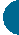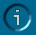Calculating Rotor RunoutComments by Gerald Kloth

One way to look at it, is if the dial indicator readings never cross zero on the dial, then the numbers are subtracted. For example, if the lowest reading is -.002" and the highest reading is -.006" (never crossed zero), then the runout is -.006 - (-.002) = -.004". A more correct way is to say that the lowest number is always subtracted from the highest number using the correct sign for each number. Highest means the most positive value. Examples:

+.001 - (-.007) = +.008
+.010 - (+.003) = +.007
-.003 - (-.016) = +.013
-.002 - (-.006) = +.004
(note that this is the same result as in my earlier example, except that the sign is correct)

Also note that the result is always positive because you can not have negative runout. I hope this doesn't confuse you. If it does, you can plot your readings on a linear scale representing the range of your indicator. The runout is the length of the line between the two readings.

-.010 .-008 -.006 -.004 -.002 0 +.002 +.004 +.006 +.008 +.010
X            X

This would be the graph for the first example. The distance between the two points = .004. This is the absolute value. It is actually good that you got the outside of the rotors running around .001" before you measure the inside face, if you use the indicator. Otherwise, you could measure, for example, .006" runout on the outside and .003" on the inside and not know if the readings are in phase or out of phase. With the outside running that good, any runout on the inside indicates that the faces are not parallel. This would certainly cause the brakes to chatter.